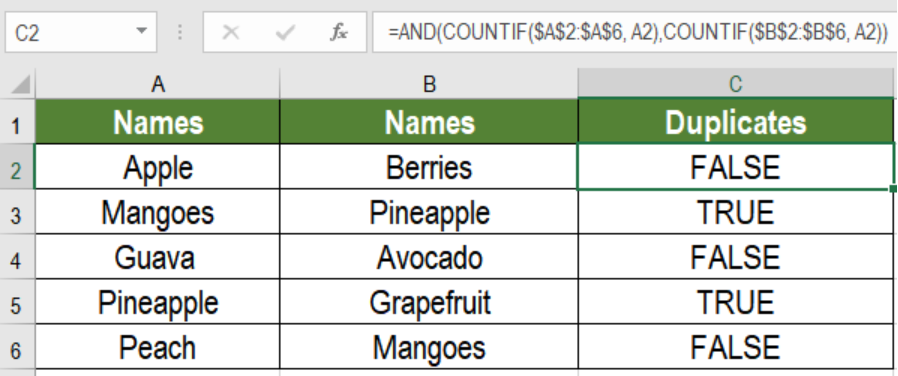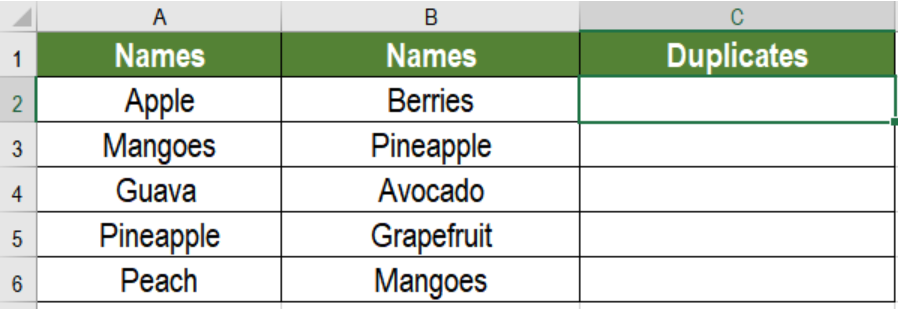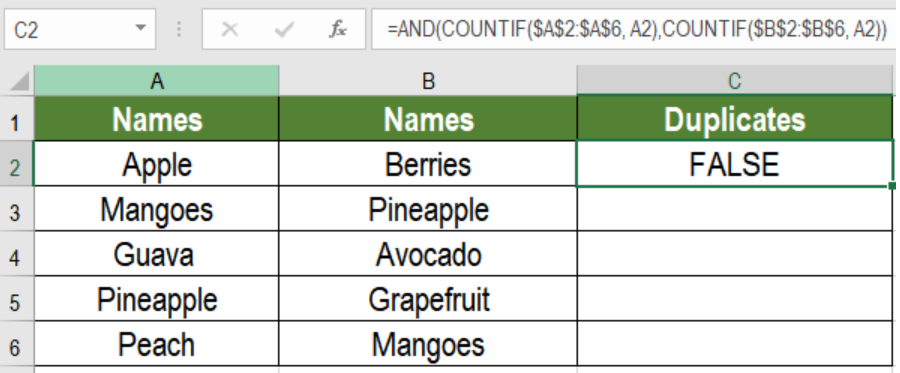Get instant live expert help with Excel or Google Sheets“My Excelchat expert helped me in less than 20 minutes, saving me what would have been 5 hours of work!”

#### Post your problem and you’ll get expert help in seconds.

Your message must be at least 40 characters
Our professional experts are available now. Your privacy is guaranteed.

# Find duplicate values in two columns

Working with big amount of data often requires us to identify duplicate values.Microsoft Excel has made finding duplicates very easy. We can combine the COUNTIF and AND functions to find duplicates between columns. In this tutorial, we will learn how to find duplicate values in two columns in Excel.Figure 1. Example of How to Find Duplicate Values in Two Columns

## Generic Formula

`=AND(COUNTIF(range1, value1),COUNTIF(range2, value1))`

## How this formula works

This formula is based on the COUNTIF function. It returns a count of all the values in both range1 and range2. The values returned by COUNTIF will either be zero (0) or a positive number in both ranges. Excel will represent the zeros as FALSE and the positive numbers as TRUE.

The two COUNTIF functions are nested inside an AND function. This will return TRUE if both counts are positive. Otherwise, it will return FALSE. The TRUE instances will represent the duplicate values in the two columns.

## Setting Up Data

The following example contains some sample fruit names. Column A and B has these names.Figure 2. The Example Data Set

To find out whether the names in column B are duplicates, we need to:

• Go to cell C2.
• Assign the formula `=AND(COUNTIF(\$A\$2:\$A\$6, A2),COUNTIF(\$B\$2:\$B\$6, A2)) `in C2.
• Press Enter.Figure 3. Applying the Formula

• Drag the formula from cells C2 to C6 using the fill handle on the bottom right.

Column C will now show TRUE for the values pineapples and mangoes which are duplicates.

Excel has made working with duplicates very simple. As we saw, combining COUNTIF and AND functions can quickly find out duplicates in two columns. However, we can  use conditional formatting to display a more visual result.

Most of the time, the problem you will need to solve will be more complex than a simple application of a formula or function. If you want to save hours of research and frustration, try our live Excelchat service! Our Excel Experts are available 24/7 to answer any Excel question you may have. We guarantee a connection within 30 seconds and a customized solution within 20 minutes.

Are you still looking for help with Conditional Formatting? View our comprehensive round-up of Conditional Formatting tutorials here.

### Did this post not answer your question? Get a solution from connecting with the expert.Another blog reader asked this question today on Excelchat:
Solution examplesYes hello Im looking for help on conditional formatting I currently have a conditional format for D9>D32 and so on from D9:S20. I need to add an if statement that references cell D4 if the first four characters are 1358 for the rule to apply can you please assist?
Solved by Z. U. in 20 minsAny rep that has orders over 500 and occurrences at zero for each month, for a 6 month period. I need a sum
Solved by C. E. in 60 minsUse the Vlookup Function to complete the "employee" column of table 2. Use "job Id" from table 2 as your lookup_value(s) and table 1 as your reference.
Solved by C. H. in 16 minsI have a list or people who are ranked in numbers from 3 to 6 I need to recognize the contents of each multiple cells and create an equivalent letter. for that value into another column. e.g. Cell E1 = 3 to show in new cell that row (H1) the letter "C" I have multiple rows with different values in column "E" Can this be done?
Solved by I. J. in 30 minsIf a cell in another sheet is populated I need a vlookup done. If the cell is not populated I need the cell to return blank.
Solved by T. D. in 60 mins## Subscribe to Excelchat.coAnother blog reader asked this question today on Excelchat: